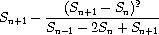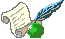...making Linux just a little more fun!

# Python Generator Tricks

By Pramode C.E.

The Python programming language's support for generators is described in PEP 255. This article demonstrates a few simple programs which make use of this feature to do some fun stuff like filtering out prime numbers, representing an `infinite' series expansion in a finite way, applying the Euler `accelerator' to make a series converge faster etc. Many of the programs which I describe here have been taken from `test_generators.py' which is available with the Python source distribution. A few ideas have been stolen from the Computer Science classic, Structure and Interpretation of Computer Programs.

## What is a Generator?

A generator is, simply put, a function which can stop whatever it is doing at an arbitrary point in its body, return a value back to the caller, and, later on, resume from the point it had `frozen' and merrily proceed as if nothing had happened. Here is a simple example:

```
from __future__ import generators

def foo():
print 'hello'
yield 1
print 'world'
yield 2

```

I am using Python 2.2 - in order to use the generator facility, a special `import' statement should be placed at the very beginning of the file. It may not be required in later versions.

Note the `yield' keyword. A function which contains a yield statement anywhere in its body is considered to be special by the Python interpreter - it is treated differently from ordinary functions. Let's see how:

```
>>> from gen1 import *
>>> a = foo()
>>> print a
<generator object at 0x8158db8>

```

We note that calling the function did not result in the function getting executed. Instead, the Python interpreter gave us a `generator object'. This is one of the implications of using the yield statement in the body of the function. Now, what do we do with this generator object?

```
>>> a.next()
hello
1
>>> a.next()
world
2
>>> a.next()
Traceback (most recent call last):
File "<stdin>" line 1, in ?
StopIteration

```

Calling a.next() resulted in the function beginning its execution - it prints hello and comes to a dead stop at the `yield' statement, returning the value 1 to the caller. The function has gone back to its caller, but its `local state' has been fully preserved. Another invocation of a.next results in the function restarting from where it had stopped earlier - it prints `world' and stops after returning the value 2 to the caller. Yet another invocation of a.next results in the function `falling off' the end - because our function is a special `generator function', this will result in an exception, StopIteration, being raised.

Let's now try running a for loop on our generator:

```
>>> a = foo()
>>> for i in a:
...    print i
...
hello
1
world
2
>>>

```

The for loop works by invoking a.next() and assigning the value obtained to i, which then gets printed. The strings 'hello' and 'world' get printed as part of the execution of `foo'. It would also be interesting to try out invoking the `list' function on the generator object - we will get a list [1,2] as the result. In both cases (for loop as well as `list'), iteration stops when the StopIteration exception is raised.

The body of a generator function should not contain a return statement of the form `return expr' - a simple `return' is allowed. The PEP discusses this and many more things. You should try running the following code:

```from __future__ import generators

def foo(n):
if (n < 3): yield 1
else: return
yield 2
```

Try running a for loop over the generator objects returned by say, foo(10) and foo(1). Also, try calling next() on these objects.

## Representing infinite sequences

Generators present us with some fun ways to manipulate infinite sequences - though some people might question their practical utility! As far as we are concerned, being fun is reason enough!

```from __future__ import generators

def foo():
i = 0
while 1:
yield i
i = i + 1
```

What we have above is the simplest possible `infinite' generator. Try calling next() on the generator object returned by calling `foo'. Give this object as an argument to a `for' loop - you will see that the loop keeps on printing numbers. If you wish Python to eat up memory, try running `list(foo())'. Try writing a more interesting function, say a Fibonacci series generator.

Here is an infinite series of alternating positive and negative terms:

1 - 1/3 + 1/5 - 1/7 + ...

This series converges to PI/4. We will write a Python generator for it.

```
def pi_series():
sum = 0
i = 1.0; j = 1
while(1):
sum = sum + j/i
yield 4*sum
i = i + 2; j = j * -1

```

Each `yield' statement keeps on returning a better approximation for PI. Test it out by calling `next' on the generator returned by invoking pi_series. We note that the series does not converge very fast.

It would be convenient to have a function which would return the first N values yielded by a generator.

```
def firstn(g, n):
for i in range(n):
yield g.next()

```

Note that the first argument to this function is a generator object. Here is what I got when I tried out `list(firstn(pi_series(), 8))':

```
[4.0, 2.666666666666667, 3.4666666666666668, 2.8952380952380956,
3.3396825396825403, 2.9760461760461765,
3.2837384837384844, 3.0170718170718178]

```

We can apply a `sequence accelerator' to convert a series of terms to a new series which converges to the original value much faster. One such accelerator, invented by Leonhard Euler, is shown below:(Sn+1) stands for the (n+1)th term, (Sn-1) for the (n-1)th term.

If Sn is the n'th term of the original sequence, then the accelerated sequence has terms as shown in the equation above.

Let's try writing a generator function which accepts a generator object and returns an `accelerated' generator object.

```
def euler_accelerator(g):
s0 = g.next() # Sn-1
s1 = g.next() # Sn
s2 = g.next() # Sn+1
while 1:
yield s2 - (sqr(s2 - s1))/(s0 - 2*s1 + s2)
s0, s1, s2 = s1, s2, g.next()

```

Here is what I got when I tried printing the first few terms of this series:

```
[3.166666666666667, 3.1333333333333337, 3.1452380952380956,
3.1396825396825401, 3.1427128427128435, 3.1408813408813416,
3.1420718170718178, 3.1412548236077655]

```
Note that the series is converging much faster! You can get the program as a whole:

## The Eratosthenes sieve

A cute idea for `filtering out' prime numbers, invented by the Alexandrian mathematician Eratosthenes, works as follows. Suppose you want to find out all prime numbers below, say, 1000. You first cancel all multiples of 2 (except 2) from a list 1..1000. Now you will cancel all multiples of 3 (except 3). 4 has already been canceled, as it is a multiple of 2. Now you will take off all multiples of 5, except 5. And so on. Ultimately, what remains in the list would be prime numbers!

Let's start with a generator which gives us all integers from `i' onwards:

```
def intsfrom(i):
while 1:
yield i
i = i + 1

```
Now let's write a generator which will eliminate all multiples of a number `n' from a sequence:
```
def exclude_multiples(n, ints):
for i in ints:
if (i % n):
yield i

```
An invocation of the generator, say, list(firstn(exclude_multiples(2, intsfrom(1)), 5)), will give us the list [1,3,5,7,9].

Now, its time for us to build our `sieve'.

```
def sieve(ints):
while 1:
prime = ints.next()
yield prime
ints = exclude_multiples(prime, ints)

```
You can get the source file containing these function definitions from here:

## Recursive Generators

Generator functions can call themselves recursively. It takes some time getting used to it. Let's try analyzing the way the following functions work:

```from __future__ import generators

def abc():
a = deff()
for i in a:
yield i
yield 'abc'

def deff():
a = ijk()
for i in a:
yield i
yield 'deff'

def ijk():
for i in (1,2,3):
yield i
yield 'ijk'

```
An invocation of abc will yield a generator object. Calling `next' on it would result in `abc' starting execution. The very first line of `abc' invokes `deff' which returns a generator object. After that, a.next() is invoked as part of the very first iteration of the for loop. This results in `deff' starting execution the same way. The body of `deff' builds a generator object by calling `ijk' and calls its `next' method as part of the for loop. This results in `ijk' starting execution and yielding 1, `deff' also yields 1, and `abc' also yields 1. Calling the `next' method (of the generator object returned by invoking `abc') two more times will result in the values 2 and 3 getting propagated up. Yet another invocation will result in the string `ijk' propagating up the call stack because the for loop in the body of `ijk' has terminated. Calling `next' again will result in the body of `ijk' terminating, with the result that the `for' loop in `deff' gets a StopIteration exception, which results in that loop terminating and the function yielding the string `deff'. Subsequent invocation of `next' will result in `abc' being returned to the top level caller. The final invocation of next (again, note that we are invoking `next' on the object returned by calling `abc') will result in the caller getting a StopIteration exception because the body of `abc' has also been executed in full.

Let's now look at Guido's binary tree example. The classical inorder traversal is coded as below:

```
def inorder(t):
if t:
for x in inorder(t.left):
yield x
yield t.dat
for x in inorder(t.right):
yield x

```
Let's think of invoking inorder on a tree with only one node (say containing data 50). Doing `for x in inorder(t.left)' is same as:
```
a = inorder(t.left)
for x in a:
....

```
Because t.left is 0, calling a.next() (which the for loop does) results in a StopIteration exception - this terminates the loop immediately. The next statement in the body is `yield t.dat' - this returns 50. The next for loop also terminates immediately because of a StopIteration. It should be easy to visualize the way the code works for more complex tree structures. Here is the source for the program - [ Listing 7 ].

## Zero crossing detector

Let's define a `signal' as a stream of positive and negative integers.

1 2 -1 -4 3 2 -3 -4 2 3 4 -2 ...

A zero-crossing detector outputs a signal which describes the zero crossings of the input signal - the resulting signal is +1 whenever the input signal changes from negative to positive, -1 whenever input signal changes from positive to negative and 0 otherwise. We shall assume that 0 is positive.

Here is the zero-cross detector:

```
def zerocross(g):
a = g.next()
b = g.next()
while 1:
yield cross_detect(a, b)
a, b = b, g.next()

```
If the signal is coming from a sensor, noise will lead to spurious zero crossings. So, we can think of `smoothing' the signal (using some form of `moving average' computation) and then detecting the zero crossings.

## Acknowledgements

Most of the code has been `lifted' from `test_generators.py', which comes with the Python source distribution. Thanks to the Python community for many hours of pleasurable code reading, and for creating the BEST programming language in the world! Thanks to the authors of SICP for making such a classic freely available on the web!I am an instructor working for IC Software in Kerala, India. I would have loved becoming an organic chemist, but I do the second best thing possible, which is play with Linux and teach programming!

Copyright © 2004, Pramode C.E.. Released under the Open Publication license unless otherwise noted in the body of the article. Linux Gazette is not produced, sponsored, or endorsed by its prior host, SSC, Inc.

Published in Issue 100 of Linux Gazette, March 2004